TitleCollege Algebra
Tutorial 54A:
SequencesLearning Objectives

 After completing this tutorial, you should be able to: Know what a sequence, term, nth term, arithmetic sequence, geometric sequence, Fibonacci sequence, finite sequence, infinite sequence, and  recursive formula are. Evaluate any term of a finite sequence. Evaluate any term of an infinite sequence. Evaluate a factorial. Write a formula of a given sequence. Work with a recursive formula.Introduction

 In this tutorial we will mainly be going over sequences.  We will start by going through some basic terminology associated with sequences.  Arithmetic and geometric sequences are special forms that are touched on lightly here, but are looked at more in depth in Tutorial 54C: Arithmetic Sequences and Series and Tutorial 54D: Geometric Sequences and Series.  We will be looking at sequences forwards and backwards.  In some cases, you will be given the formula for the nth term, and you will need to come up with the term and in other cases you are shown the pattern of the terms in the sequence and you will need to come up with the formula.  Once you are able to go back and forth, then that means you have sequences down.  Enough chit chat, let's get started.Tutorial

 Sequence

 In general, a sequence is an ordered arrangement of numbers, figures, or objects. Sequences of math are a string of numbers that are tied together with some sort of consistent rule, or set of rules, that determines the next number in the sequence.

 Terms

 The terms of a sequence are the output values or dependent variables.

 nth or general term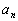represents the nth term of a sequence. a represents the functional or output value and n represents the input value of term number.  For example,represents the fifth term of the sequence.

 Arithmetic Sequence

 An arithmetic sequence is a sequence such that each successive term is obtained from the previous term by addition or subtraction of a fixed number called a difference. The sequence 4, 7, 10, 13, 16, ... is an example of an arithmetic sequence - the pattern is that we are always adding a fixed number of three to the previous term to get to the next term. Be careful that you don't think that every sequence that has a pattern in addition is arithmetic. It is arithmetic if you are always adding the SAME number each time. For a more in depth look at arithmetic sequences, feel free to go to Tutorial 54C: Arithmetic Sequences and Series.

 Geometric Sequence

 A geometric sequence is a sequence such that each successive term is obtained from the previous term by multiplying by a fixed number called a ratio. The sequence 5, 10, 20, 40, 80, .... is an example of a geometric sequence.  The pattern is that we are always multiplying by a fixed number of 2 to the previous term to get to the next term. Be careful that you don't think that every sequence that has a pattern in multiplication is geometric. It is geometric if you are always multiplying by the SAME number each time. For a more in depth look at geometric sequences, feel free to go to Tutorial 54D: Geometric Sequences and Series.

 Fibonacci Sequence

 A basic Fibonacci sequence is when two numbers are added together to get the next number in the sequence. 1, 1, 2, 3, 5, 8, 13, .... is an example of a Fibonacci sequence where the starting numbers (or seeds) are 1 and 1, and we add the two previous numbers to get the next number in the sequence.

 Finite Sequence

 A finite sequence is a sequence whose domain consists of the set {1, 2, 3, ... n} or in other words the first n positive integers.

 Infinite Sequence

 An infinite sequence is a sequence whose domain consists of the set {1, 2, 3, ...} or in other words all positive integers.

 Factorial !

 The factorial symbol is the exclamation point: ! So if I wanted to write 7 factorial it would be written as 7!. In general, n! = n(n - 1)(n - 2)(n - 3)...(1) Most, (if not all), of you will have a factorial key on your calculator.  It looks like this: ! If you have a graphing calculator, it will be hidden under the MATH menu screen and then select your Probability screen - there you should find ! 0!  Has a special definition attached with it.  0! = 1Example 1: Find all of the terms of the finite sequence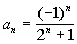,    1 < n < 5

 Basically, to find the nth term of a sequence works in the same fashion as function notation.  If you want to find the 3rd term, you are looking for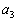, which means you plug in 3 for n into the given function. So, what are we going to plug in for n to find the 1st term?  If you said 1, give yourself a pat on the back.  What about the 2nd term?  I hope you said you would plug in 2 for n.   Since we have to go from 1 < n < 5, this means we need to find 5 terms and we will be plugging in 1, 2, 3, 4, and 5 for n. Let's see what we get: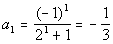*1st term, n = 1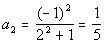*2nd term, n = 2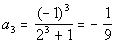*3rd term, n = 3*4th term, n = 4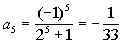*5th term, n = 5

 Note how we had -1 raised to n, which changes value, and the signs of the terms alternated. The five terms of this sequence are -1/3, 1/5, -1/9, 1/17, and -1/33.Example 2: Find the first six terms and the fifteenth term of the infinite sequence with the nth term of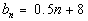.

 Note how there is no bound for n like there was in example 1.  This means the sequence goes on and on or in other words it is an infinite sequence.  We still approach finding terms the same way we did in example 1.  n is our term number and we plug the term number into the function to find the value of the term. Let's see what we get for our first six terms: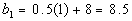*1st term, n = 1*2nd term, n = 2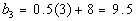*3rd term, n = 3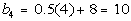*4th term, n = 4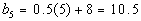*5th term, n = 5*6th term, n = 6

 The first six terms are 8, 8.5, 9, 9.5, 10, 10.5, and 11. Note how each term went up by 0. 5 from the previous term. Now let's check out the fifteenth term: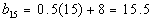*15th term, n = 15

 The fifteenth term is 15.5.Example 3: Find the first six terms and the tenth term of the infinite sequence with the nth term of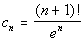.

 This function contains a factorial. Let's see what we get for our first six terms: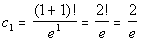*1st term, n = 1*2nd term, n = 2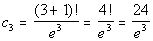*3rd term, n = 3*4th term, n = 4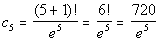*5th term, n = 5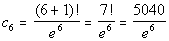*6th term, n = 6

 Now let's check out the tenth term: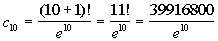*10th term, n = 10Example 4: Write a formula for the nth term of the infinite sequence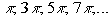.

Let's take a look at what is happening here:

When n = 1, thenn = 2, then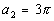n = 3, then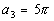n = 4, then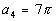an so forth.

Something that is always constant is that each term contains.

It also looks like it goes up by odd numbers starting with 1.  What we need to do is to think about what the relationship between n and the odd number (the part that changes) is:

 When n is 1, the odd number is 1. When n is 2, the odd number is 3. When n is 3, the odd number is 5. When n is 4, the odd number is 7.

What do you think the relationship is?
It looks like the number is always one less than twice n.  In other words, the number is 2n - 1.

So the formula for the nth term is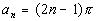.

Sometimes you have to play around with it before you get it just right.  You can always check it by putting in the n values and seeing if you get the given sequence.  This one does check.Example 5: Write a formula for the nth term of the infinite sequence -1, 8, -27, 64, ....

Let's take a look at what is happening here:

This time there isn’t anything constant, but there are two things that change.

First let's look at the alternating signs:

 For it to have alternating signs, we need to have (-1) raised to a power that changes.  This means n, the term number is involved. The first term is negative, the second term is positive, the third negative, the fourth positive and so forth. When n is odd (1, 3, 5, ...), then the term is negative. When n is even (2, 4, 6, ...), then the term is positive.  So do you think we are going to have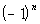or. If you saidyou are correct!!!  If n is odd, then this term will be negative.  If n is even, then this term will be positive.

Forgetting about the negative signs for a moment, we also have 1, 8, 27, 64.

Again we need to figure out the relationship between n and the term itself:

 When n is 1, the number is 1. When n is 2, the number is 8. When n is 3, the number is 27. When n is 4, the number is 64.

What do you think the relationship is?
It looks like the number is always n cubed.

Putting it together, the formula for the nth term is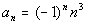.

Sometimes you have to play around with it before you get it just right.  You can always check it by putting in the n values and seeing if you get the given sequence.

This one does check.

 Recursive Formulas

 In a recursive formula, the nth term of the sequence is a function of or has a relationship with the previous term.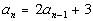is an example of a recursive formula, because in order to get the nth term you need to take 2 times the term before it and then add 3.Example 6: Find the first three terms and the fifth term of the infinite sequence given by the recursive formula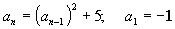.

 We are giving the first term,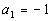.  Using that we can find the second term and so forth. Let's see what we get for our first three terms: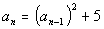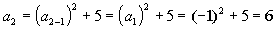*2nd term, n = 2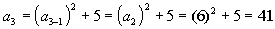*3rd term, n = 3

 Since this is a recursive formula, in order to the fifth term, we need to find the fourth term: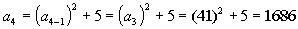*4th term, n = 4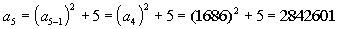*5th term, n = 5Practice Problems

 These are practice problems to help bring you to the next level.  It will allow you to check and see if you have an understanding of these types of problems. Math works just like anything else, if you want to get good at it, then you need to practice it.  Even the best athletes and musicians had help along the way and lots of practice, practice, practice, to get good at their sport or instrument.  In fact there is no such thing as too much practice. To get the most out of these, you should work the problem out on your own and then check your answer by clicking on the link for the answer/discussion for that  problem.  At the link you will find the answer as well as any steps that went into finding that answer.Practice Problem 1a: Find all of the terms of the finite sequence.

 1a.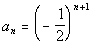;    1 < n < 5 (answer/discussion to 1a)Practice Problem 2a: Find the first five terms and the twelfth term of the infinite sequence.

 2a.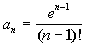(answer/discussion to 2a)Practice Problem 3a: Write a formula for the nth term of given the infinite sequence.

 3a.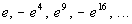(answer/discussion to 3a)Practice Problem 4a: Find the first three terms and the fifth term of the infinite sequence given by the recursive formula.

 4a.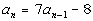;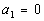(answer/discussion to 4a)Need Extra Help on these Topics?

 There were no good websites found to help us with the topics on this page.   Go to Get Help Outside the Classroom found in Tutorial 1: How to Succeed in a Math Class for some more suggestions.

Last revised on May 16, 2011 by Kim Seward.